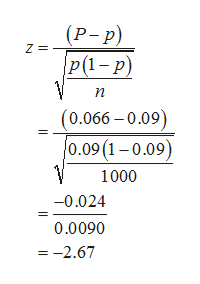It was determined that the percentage of a's in the English language in the 1800s was 9%. A random sample of 1000 letters from a current newspaper contained 66 a's. Test the hypothesis that the proportion of a's has changed in modern times, using the 0.05 level of significance. Treat the newspaper as a random sample of all letters used.

Question

It was determined that the percentage of a's in the English language in the 1800s was 9%. A random sample of 1000 letters from a current newspaper contained 66 a's. Test the hypothesis that the proportion of a's has changed in modern times, using the 0.05 level of significance. Treat the newspaper as a random sample of all letters used.

Step 1

It is given that the population proportion; p is 9% (=0.09). In the survey, it is found that 66 of 1000 letters from a current newspaper. Let p be the sample proportion

The null and alternative hypotheses are given below.

Step 2

The sample proportion, P is calculated below.

Step 3

The test statistic is given and...help_outlineImage Transcriptionclose(Р-р) P(1- р) Z п (0.066-0.09) 0.09 (1-0.09) 1000 -0.024 0.0090 =-2.67 fullscreen

Want to see the full answer?

See Solution

Want to see this answer and more?

Our solutions are written by experts, many with advanced degrees, and available 24/7

See Solution
Tagged in

Statistics